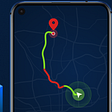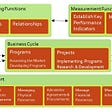# Creating a simple Bank Management System using Python

1. Importing the libraries
`import pickleimport osimport pathlib`
`class Account :    accNo = 0    name = ''    deposit=0    type = ''`
`def createAccount(self):        self.accNo= int(input("Enter the account no : "))        self.name = input("Enter the account holder name : ")        self.type = input("Ente the type of account [C/S] : ")        self.deposit = int(input("Enter The Initial amount(>=500 for Saving and >=1000 )for current"))        print("\n\n\nAccount Created")`
`def showAccount(self):        print("Account Number : ",self.accNo)        print("Account Holder Name : ", self.name)        print("Type of Account",self.type)        print("Balance : ",self.deposit)`
`def modifyAccount(self):        print("Account Number : ",self.accNo)        self.name = input("Modify Account Holder Name :")        self.type = input("Modify type of Account :")        self.deposit = int(input("Modify Balance :"))`
`def depositAmount(self,amount):        self.deposit += amount    def withdrawAmount(self,amount):        self.deposit -= amount`
`def report(self):        print(self.accNo, " ",self.name ," ",self.type," ", self.deposit)`
`def getAccountNo(self):        return self.accNo    def getAcccountHolderName(self):        return self.name    def getAccountType(self):        return self.type    def getDeposit(self):        return self.deposit`
`def writeAccount():    account = Account()    account.createAccount()    writeAccountsFile(account)`
`def displayAll():    file = pathlib.Path("accounts.data")    if file.exists ():        infile = open('accounts.data','rb')        mylist = pickle.load(infile)        for item in mylist :            print(item.accNo," ", item.name, " ",item.type, " ",item.deposit )        infile.close()    else :        print("No records to display")`
`def displaySp(num):    file = pathlib.Path("accounts.data")    if file.exists ():        infile = open('accounts.data','rb')        mylist = pickle.load(infile)        infile.close()        found = False        for item in mylist :            if item.accNo == num :                print("Your account Balance is = ",item.deposit)                found = True    else :        print("No records to Search")    if not found :        print("No existing record with this number")`
`def depositAndWithdraw(num1,num2):    file = pathlib.Path("accounts.data")    if file.exists ():        infile = open('accounts.data','rb')        mylist = pickle.load(infile)        infile.close()        os.remove('accounts.data')        for item in mylist :            if item.accNo == num1 :                if num2 == 1 :                    amount = int(input("Enter the amount to deposit : "))                    item.deposit += amount                    print("Your account is updted")                elif num2 == 2 :                    amount = int(input("Enter the amount to withdraw : "))                    if amount <= item.deposit :                        item.deposit -=amount                    else :                        print("You cannot withdraw larger amount")else :        print("No records to Search")    outfile = open('newaccounts.data','wb')    pickle.dump(mylist, outfile)    outfile.close()    os.rename('newaccounts.data', 'accounts.data')`
`def deleteAccount(num):    file = pathlib.Path("accounts.data")    if file.exists ():        infile = open('accounts.data','rb')        oldlist = pickle.load(infile)        infile.close()        newlist = []        for item in oldlist :            if item.accNo != num :                newlist.append(item)        os.remove('accounts.data')        outfile = open('newaccounts.data','wb')        pickle.dump(newlist, outfile)        outfile.close()        os.rename('newaccounts.data', 'accounts.data')`
`def modifyAccount(num):    file = pathlib.Path("accounts.data")    if file.exists ():        infile = open('accounts.data','rb')        oldlist = pickle.load(infile)        infile.close()        os.remove('accounts.data')        for item in oldlist :            if item.accNo == num :                item.name = input("Enter the account holder name : ")                item.type = input("Enter the account Type : ")                item.deposit = int(input("Enter the Amount : "))outfile = open('newaccounts.data','wb')        pickle.dump(oldlist, outfile)        outfile.close()        os.rename('newaccounts.data', 'accounts.data')`
`def writeAccountsFile(account) :file = pathlib.Path("accounts.data")    if file.exists ():        infile = open('accounts.data','rb')        oldlist = pickle.load(infile)        oldlist.append(account)        infile.close()        os.remove('accounts.data')    else :        oldlist = [account]    outfile = open('newaccounts.data','wb')    pickle.dump(oldlist, outfile)    outfile.close()    os.rename('newaccounts.data', 'accounts.data')`
`ch=''num=0intro()while ch != 8:    #system("cls");    print("\tMAIN MENU")    print("\t1. NEW ACCOUNT")    print("\t2. DEPOSIT AMOUNT")    print("\t3. WITHDRAW AMOUNT")    print("\t4. BALANCE ENQUIRY")    print("\t5. ALL ACCOUNT HOLDER LIST")    print("\t6. CLOSE AN ACCOUNT")    print("\t7. MODIFY AN ACCOUNT")    print("\t8. EXIT")    print("\tSelect Your Option (1-8) ")    ch = input()    #system("cls");if ch == '1':        writeAccount()    elif ch =='2':        num = int(input("\tEnter The account No. : "))        depositAndWithdraw(num, 1)    elif ch == '3':        num = int(input("\tEnter The account No. : "))        depositAndWithdraw(num, 2)    elif ch == '4':        num = int(input("\tEnter The account No. : "))        displaySp(num)    elif ch == '5':        displayAll();    elif ch == '6':        num =int(input("\tEnter The account No. : "))        deleteAccount(num)    elif ch == '7':        num = int(input("\tEnter The account No. : "))        modifyAccount(num)    elif ch == '8':        print("\tThanks for using bank managemnt system")        break    else :        print("Invalid choice")ch = input("Enter your choice : ")`

--

--

--

## More from Yeshwant Kumar

Love podcasts or audiobooks? Learn on the go with our new app.

## GitHub CLI Tool ⚒## Develop Location Based Applications with Huawei Mobile Services — 2## Using Enterprise Architecture to support strategic business decisions: A Case Study## App Store Transitions## Architecture Learnings Part #3 {What is in a Domain?}## 3 Must-Know Metrics For Data Analysts To Understand Customer Buying Behavor## How to Extract Amazon Products Using (ASINs)?## Best of all time? — An analysis of NBA Raptor player data using databricks